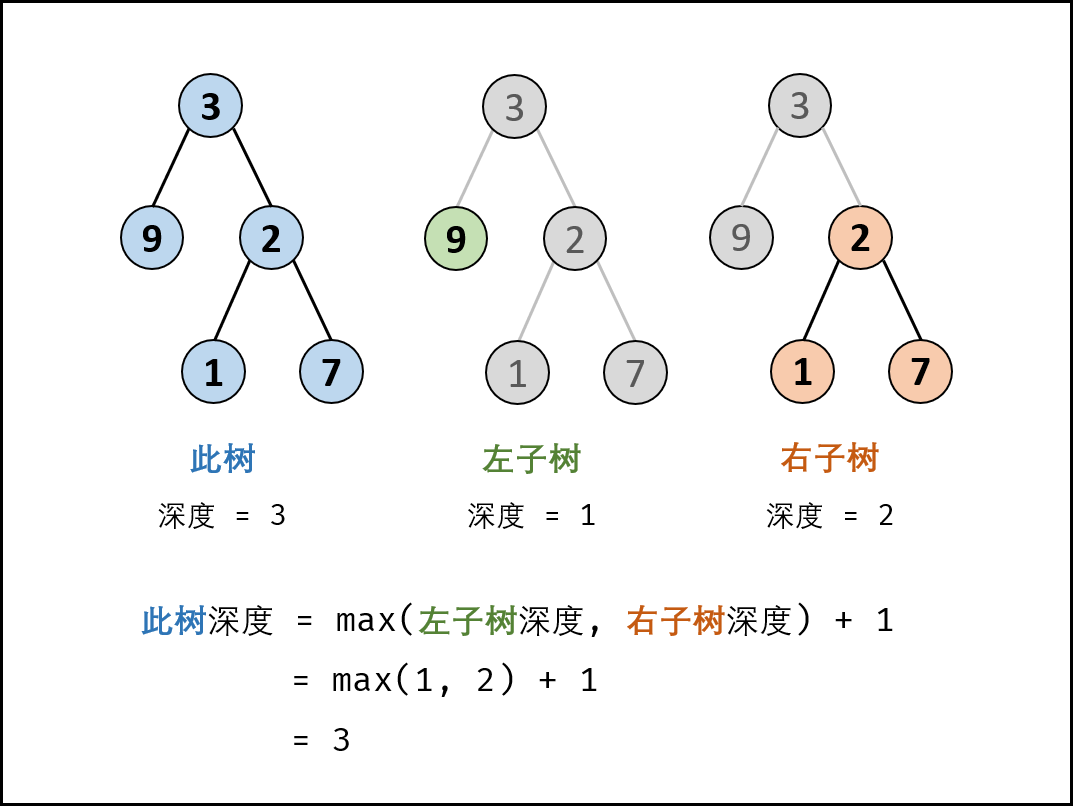# 【LeetCode】 35 平衡二叉树

## 题目

	3
/ \
9  20
/  \
15   7


  	   1
/ \
2   2
/ \
3   3
/ \
4   4


• 1 <= 树的结点个数 <= 10000

## 思路## 代码

class Solution {
public boolean isBalanced(TreeNode root) {
return recur(root) != -1;
}

private int recur(TreeNode root) {
if (root == null) return 0;
int left = recur(root.left);
if(left == -1) return -1;
int right = recur(root.right);
if(right == -1) return -1;
return Math.abs(left - right) < 2 ? Math.max(left, right) + 1 : -1;
}
}